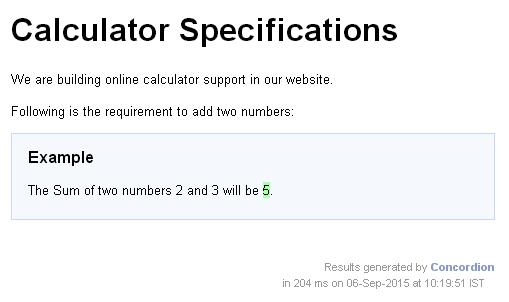# Concordion - assertEquals Command

Concordion assertEquals command is used to check Java bean property or method result against a specified value.

Consider the following requirement −

```The sum of two numbers 2 and 3 will be 5.
```

If we want the numbers 2 and 3 to be as parameters and pass them to sum function as parameter so that it can be verified against the result as 5 returned by the system then we can use concordion:assertEquals command within span tag around the sum function.

```<p>The Sum of two numbers <span concordion:set="#firstNumber">2</span>
and  <span concordion:set="#secondNumber">3</span> will be
<span concordion:assertEquals="sum(#firstNumber, #secondNumber)">5</span>.</p>
```

When Concordion parses the document, it will set a temporary variable #firstNumber to be the value "2" and #secondNumber to be the value "3" using set command and then call the sum() method with parameters as #firstNumber and #secondNumber and check that the result is equal to "5" using the assertEquals command.

### Example

Let us have working Eclipse IDE in place and follow the following steps to create a Concordion application −

Step Description
1 Create a project with a name concordion and create a package com.tutorialspoint under the src folder in the created project.
2 Add required Concordion libraries using Add External JARs option as explained in the Concordion - First Application chapter.
3 Create Java class System under the com.tutorialspoint package.
4 Create Fixture class SystemFixture under the specs.tutorialspoint package.
5 Create Specification html System.html under the specs.tutorialspoint package.
6 The final step is to create the content of all the Java files and specificiation file and run the application as explained below.

Here is the content of the System.java file −

```package com.tutorialspoint;
public class System {
public int sum(int firstNumber, int secondNumber) {
return firstNumber + secondNumber;
}
}
```

Following is the content of the SystemFixture.java file −

```package specs.tutorialspoint;

import org.concordion.integration.junit4.ConcordionRunner;
import org.junit.runner.RunWith;
import com.tutorialspoint.System;

@RunWith(ConcordionRunner.class)

public class SystemFixture {
System system = new System();
public int sum(int firstNumber, int secondNumber) {
return system.sum(firstNumber, secondNumber);
}
}
```

Following is the content of the System.html file −

```<html xmlns:concordion = "http://www.concordion.org/2007/concordion">
<link href = "../concordion.css" rel = "stylesheet" type = "text/css" />

<body>
<h1>Calculator Specifications</h1>
<p>We are building online calculator support in our website.</p>
<p>Following is the requirement to add two numbers:</p>

<div class = "example">
<h3>Example</h3>
<p>The Sum of two numbers <span concordion:set = "#firstNumber">2</span>
and  <span concordion:set = "#secondNumber">3</span> will be
<span concordion:assertEquals = "sum(#firstNumber, #secondNumber)">5</span>.</p>
</div>

</body>

</html>
```

Once you are done with creating source and specification files, let us run the application as JUnit Test. If everything is fine with your application, then it will show the following result −

```C:\DOCUME~1\ADMINI~1\LOCALS~1\Temp\concordion\specs\tutorialspoint\System.html
Successes: 1, Failures: 0
```

System.html is the output of Concordion test run.Successes: 1, Failures: 0